# Bisection Method Example: Hand Solution and Python Code

Find the solution of the following equation using the bisection method: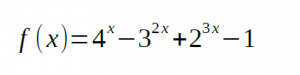Write a Python 3 code for this method.

Solution

Hand Solution

Let’s choose the initial values of x so that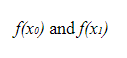have different signs: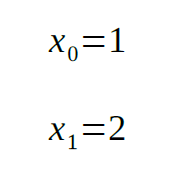Plugging the above values into the equation, we get: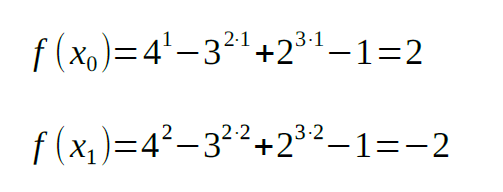The next value of x is: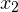value into the equation: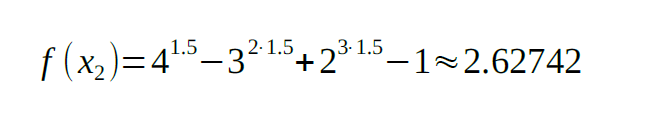As the signs of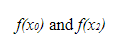are the same, we will replace the x0 value with x2 and repeat the above steps: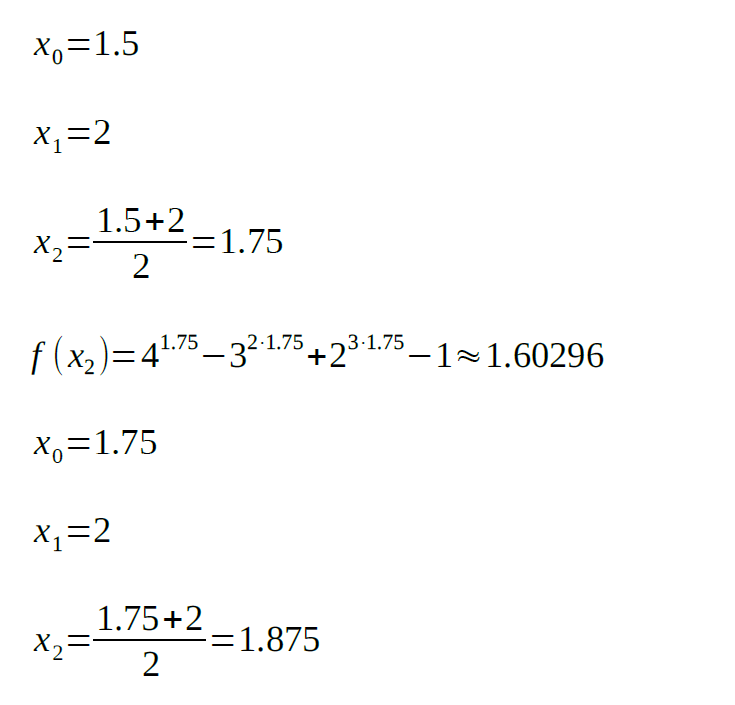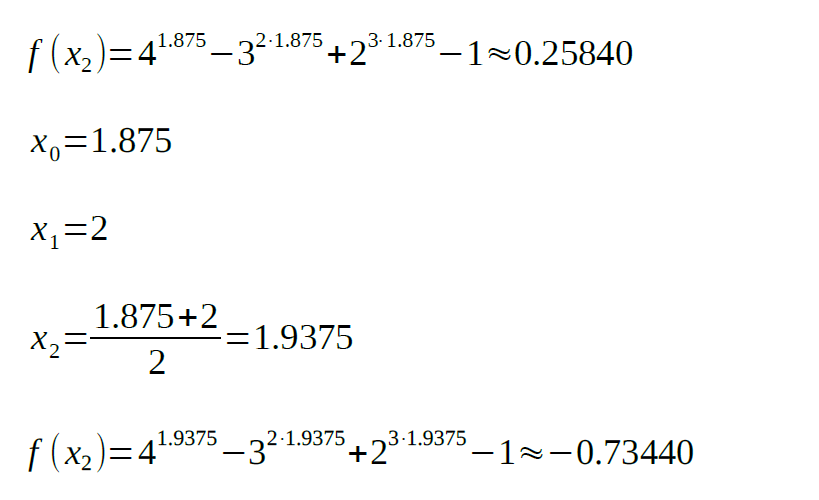Now, we will replace the x1 value with x2, as the signs of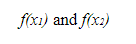are the same: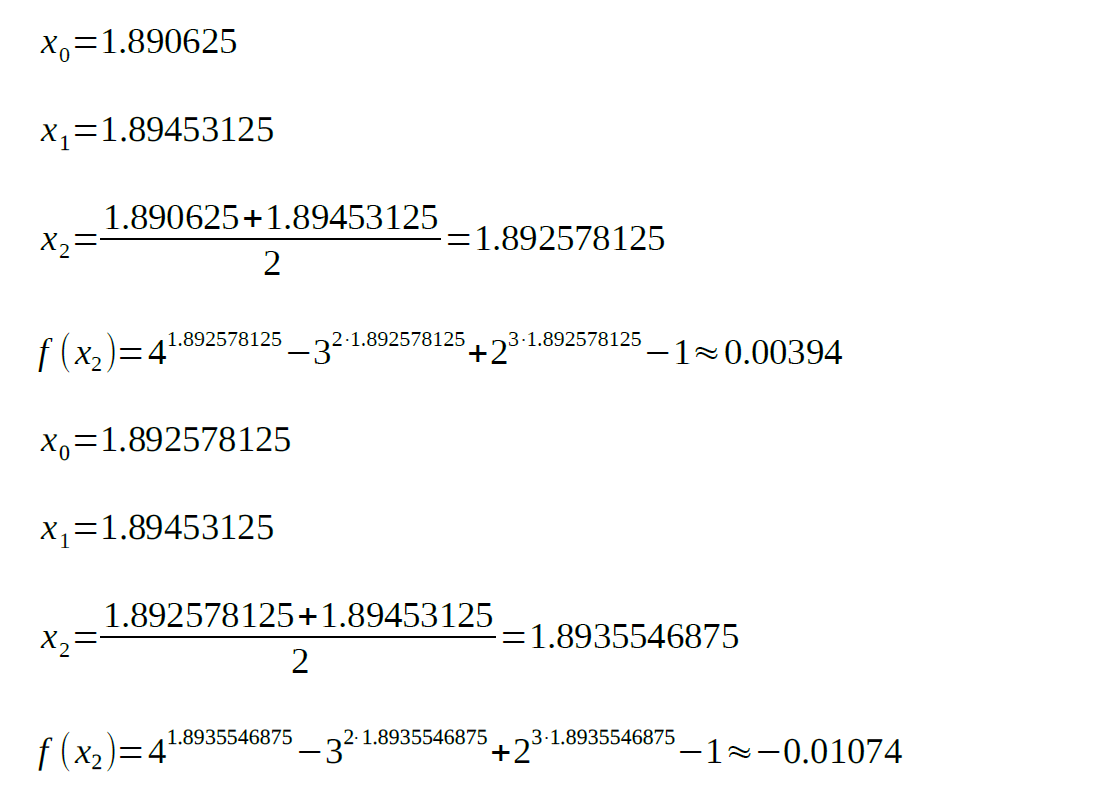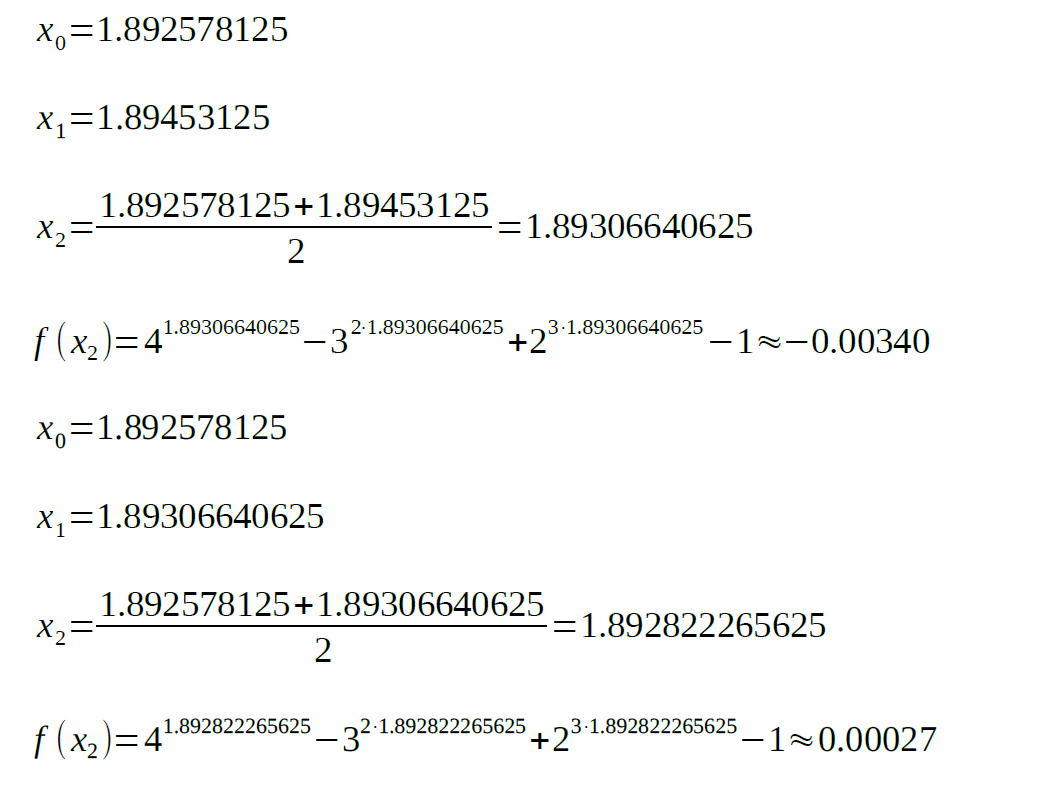As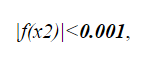the root of the equation is approximately equal to 1.89282.

Python 3 Code

Let’s define a function for our equation first:Now, we will write a function for defining the sign: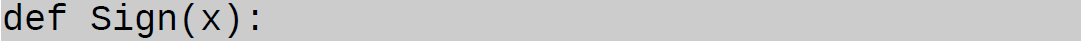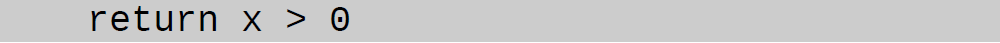This is our main function for finding the root of the equation. As we must choose two initial values for x, our function should accept two initial parameters: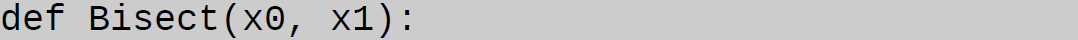For simplification:And let’s repeat the above steps in a while loop. In order to prevent infinite loop, it’s important to add an exit condition. In our case we will check if the absolute value of y is larger than the 0.001 threshold. To decide what x value should be replaced, the sign check statement will be written as follows: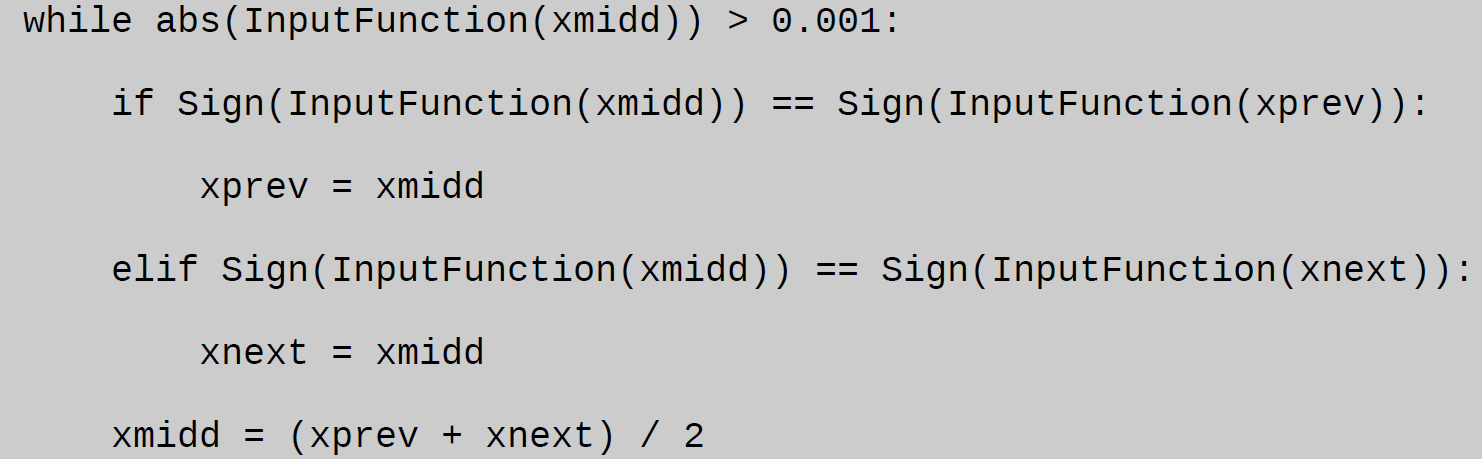At last, the found x value is returned: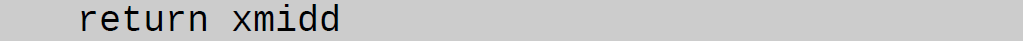The entire code: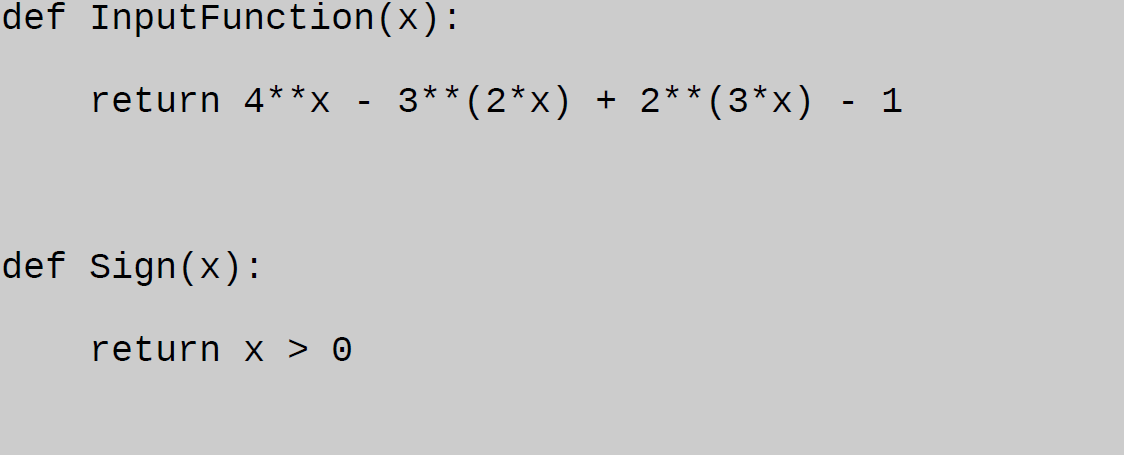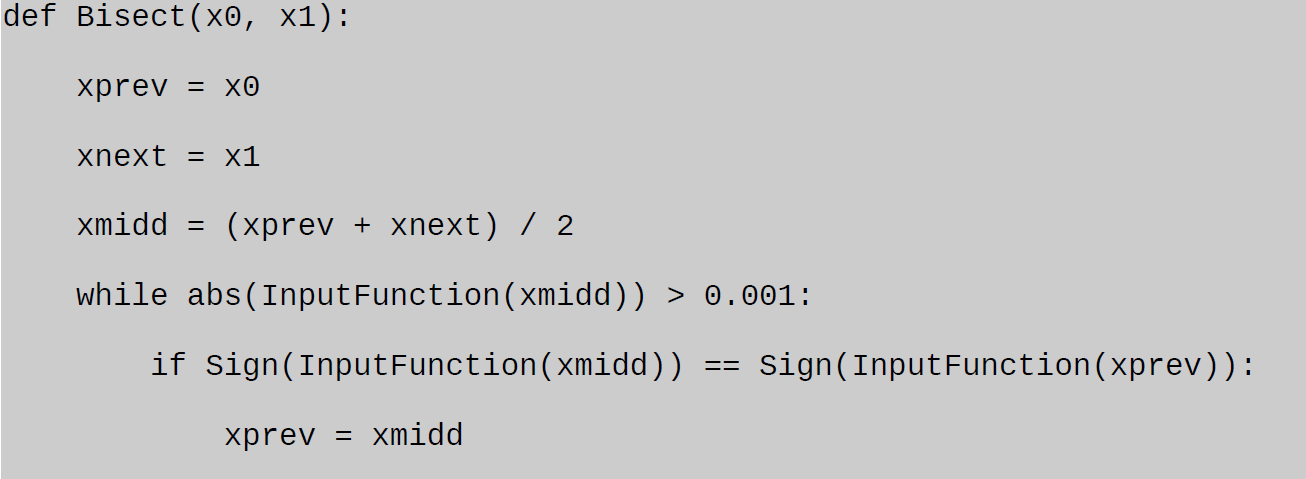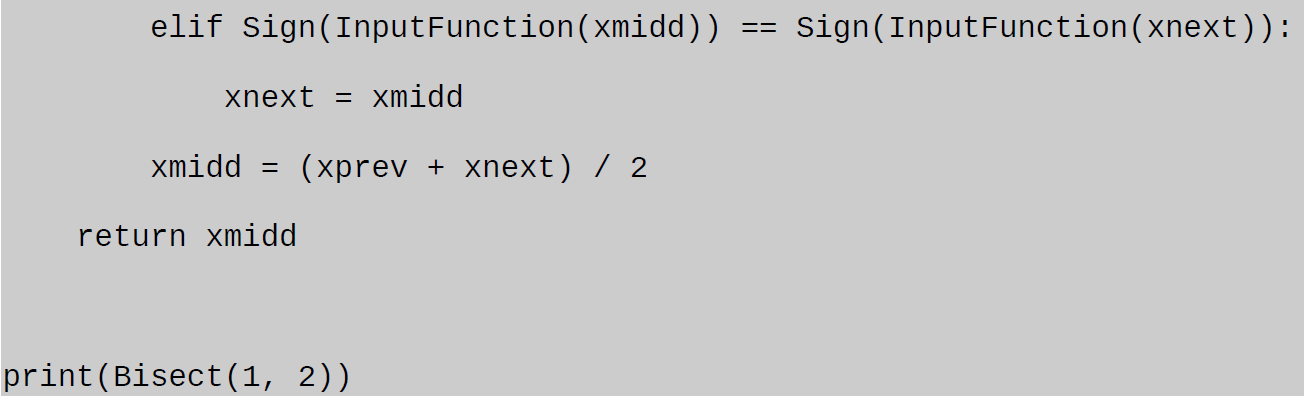The bisection method is one of the root-finding methods for continuous functions. If you want to become an expert at mathematics, you should carefully check our bisection method example and learn more about it.

Our expert has provided two solutions for the equation: hand solution and Python code. You can use them as an example for your assignments. In case you have difficulties with mathematics or don’t have time for your homework, you can apply to our service. We work 24/7 and help students with various assignments. One of our technical writers will help you deal with the bisection method in Python or any other homework assignment.

AssignmentShark is a team of professionals with one common purpose – to make the life of students easier by providing math assignment help. We don’t want you to waste your time in college on endless assignments or obligatory disciplines! Do what you want, and we’ll take care of your homework.